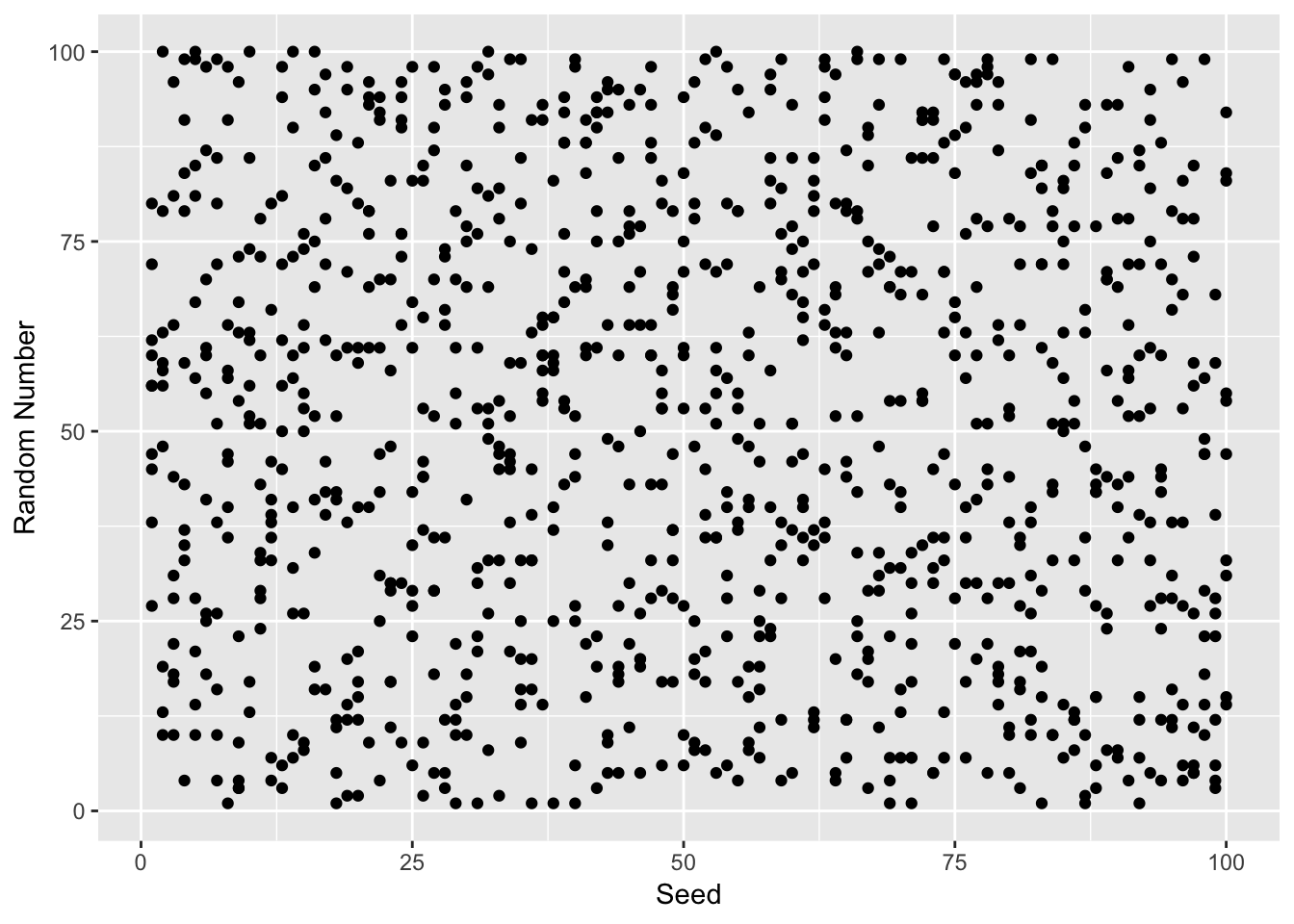# Random Numbers and Seeds in R

To explore how R generates randome numbers, we will use the rnorm function. This function draws a random number from a normal distribution with a mean = 0 and standard deviation = 1 (though these can be changed with the mean and sd parameters). With n = 1 we will get two random numbers.

rnorm(n = 1)
##  -0.0370592
rnorm(n = 1)
##  0.1957395

Each time you run the command you will get a different number. The set.seed function will sets a seed to the random number generator so that each subsequent run will produce the same number.

set.seed(2112); rnorm(n = 1)
##  0.9243372
set.seed(2112); rnorm(n = 1)
##  0.9243372

Setting a different seed results in a different number.

set.seed(2113); rnorm(n = 1)
##  0.5499032

What are seeds?

Computers are actually bad at random events. However, there are good algorithms that mimic random processes. These algorithms work by starting with some initial value, a seed, and executing a complex algorithm that approximates randomozation. The seed is often set to the current time in miliseconds. To visualize the ramdom process, we will use the sample function to randomly select a number between 1 and 100. We will consider the ouput for the first 1,000 seeds.

random_numbers <- integer(1000)
for(i in seq_len(length(random_numbers))) {
set.seed(i)
random_numbers[i] <- sample(1:100, size = 1)
}
library(ggplot2)
ggplot(data.frame(x = 1:100, y = random_numbers),
aes(x = x, y = y)) +
geom_point() +
xlab('Seed') + ylab('Random Number')